# LCR Meter vs. Multimeter: What’s the Difference?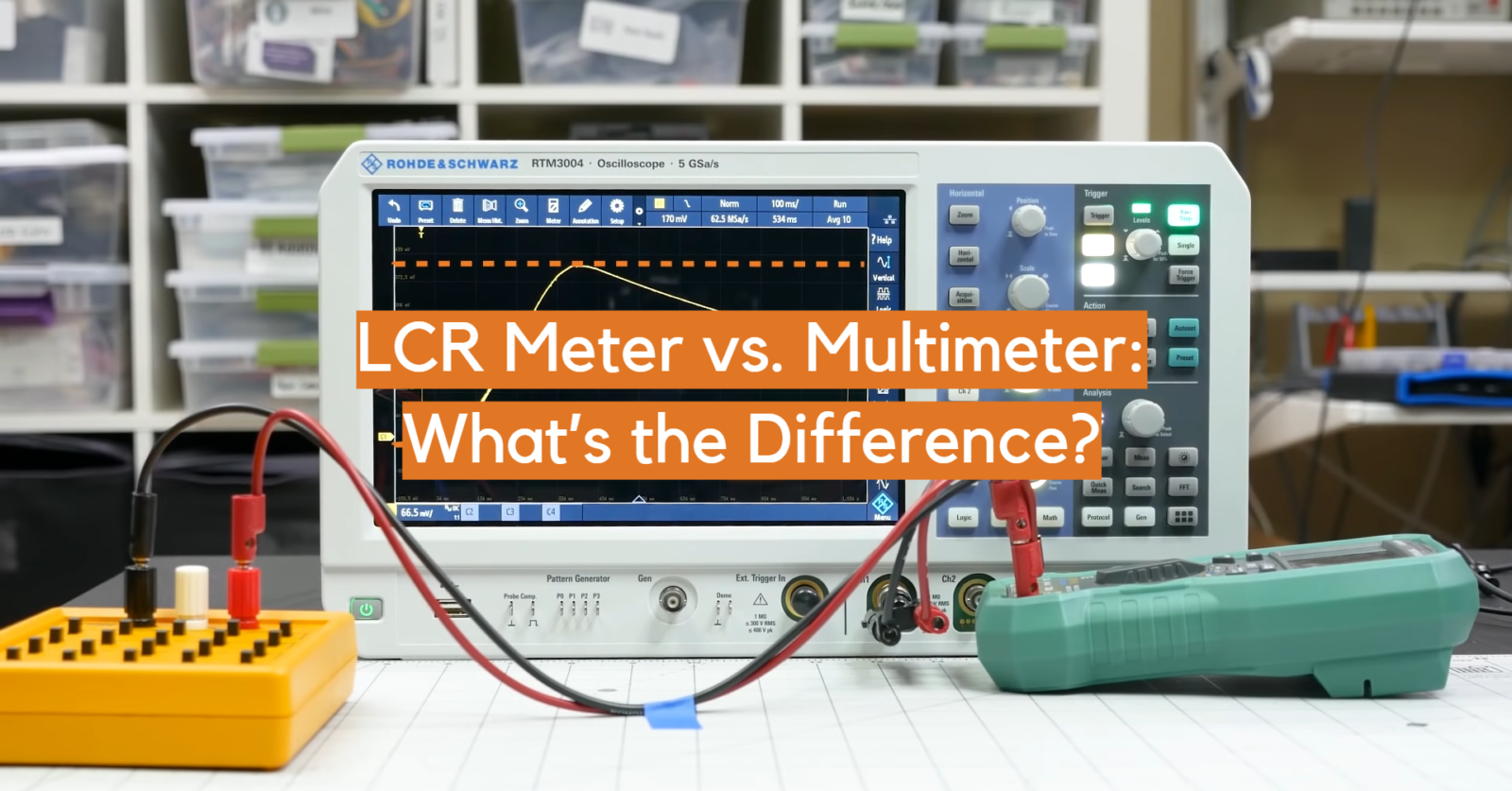When it comes to electronics and electrical testing, two common tools used by technicians are LCR meters and multimeters. But what sets them apart?

An LCR meter is specifically designed to measure inductance (L), capacitance (C), and resistance (R) in an electronic circuit. On the other hand, a multimeter can also measure these components, but it is able to perform a wider range of measurements such as voltage, current, and even temperature.

A multimeter is a versatile and cost-effective option for general electronic testing, but for more precise measurements of LCR components, an LCR meter is the preferred tool.

So why use an LCR meter instead of a multimeter for these measurements? The main difference is accuracy and precision. Multimeters have lower accuracy and precision when it comes to measuring components like inductors and capacitors, whereas an LCR meter is designed specifically for those measurements.

In this article, we’ll dive deeper into the capabilities and applications of both LCR meters and multimeters.

## Multimeter Design

### Display

A multimeter typically has a digital or analog display. It can measure voltage, current, and resistance.

### Components

A multimeter has different probes and ports for measuring various electrical properties. It can also measure continuity, dwell, and diode testing.

### Button Panel

A multimeter has a button panel with buttons to switch between the different measurement modes and settings.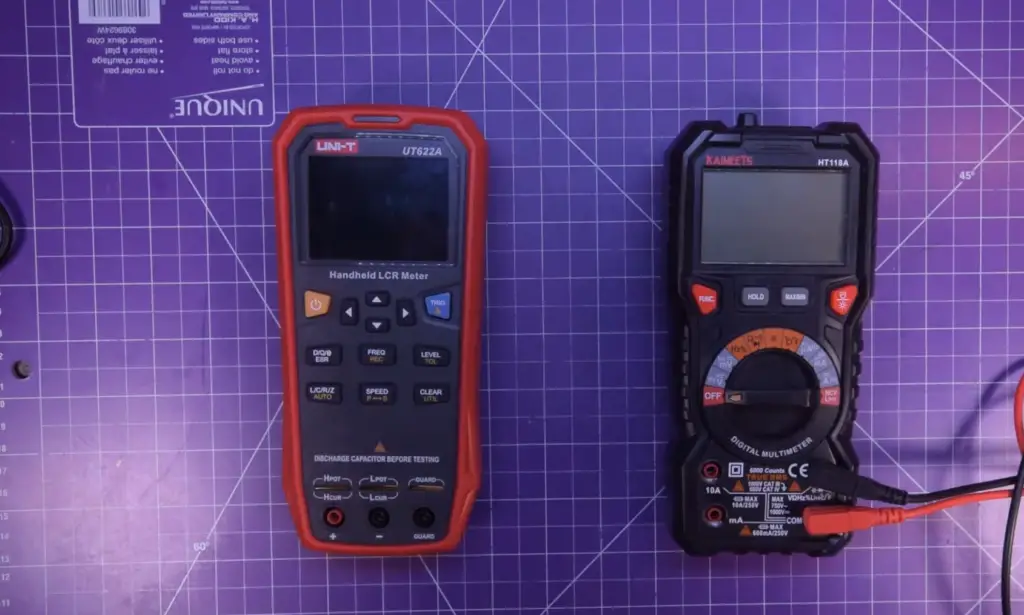### Dial

Some multimeters also have a dial to switch between measurement ranges.

### Input Jacks

A multimeter has input jacks for the different probes to connect to. Also, a multimeter can measure AC and DC properties.

## Multimeter Uses

### Checking The Voltage Of Batteries

A multimeter may check for voltage, current, and resistance in a circuit. However, it is not as accurate as an LCR meter when measuring the latter two properties.

### Examining Power Outlets

A multimeter may also be used to check for proper function in power outlets, by measuring the voltage and current flow.

### Testing A Fuse

A multimeter can also test a fuse to see if it is functioning properly.

### Locating A Bad Switch Or Wire

A multimeter can also be used to locate a faulty switch or wire in a circuit.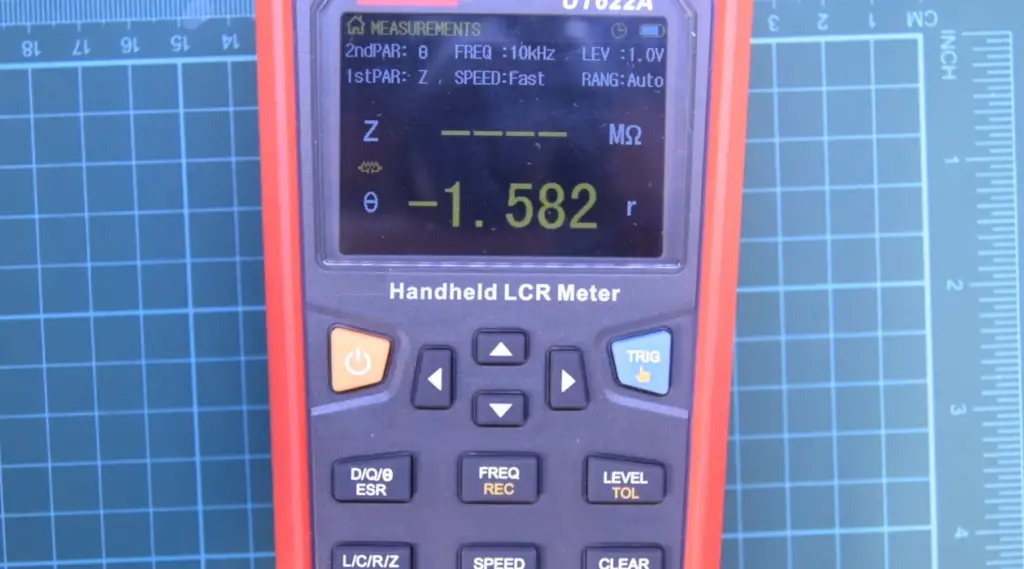## LCR Meter Design

### On/Off Switch

An average LCR meter is designed with an on/off switch to activate or deactivate the device. This feature allows you to conserve battery life and avoid accidents while not in use.

### Test Frequency Selection

LCR meters typically allow the user to select a test frequency, ranging from low frequencies (such as 100Hz) to high frequencies (such as 1MHz). The ability to adjust test frequency is important because it can affect the measurement results of certain components.

### AC and DC Testing Capabilities

LCR meters are designed with both AC and DC testing capabilities, making them suitable for measuring a variety of components such as capacitors, inductors, and resistors.

### Function Selector

LCR meters have a function selector that allows the user to choose what type of measurement they want to conduct. This could include measuring capacitance, inductance, resistance, or a combination of these qualities (impedance).

### Display

LCR meters have a display screen, typically with multiple lines, that shows the measurement results. Some models also feature a graphical display for a visual representation of the data.

### Range Selector

LCR meters have a range selector to adjust the measurement range, allowing for readings that are more accurate.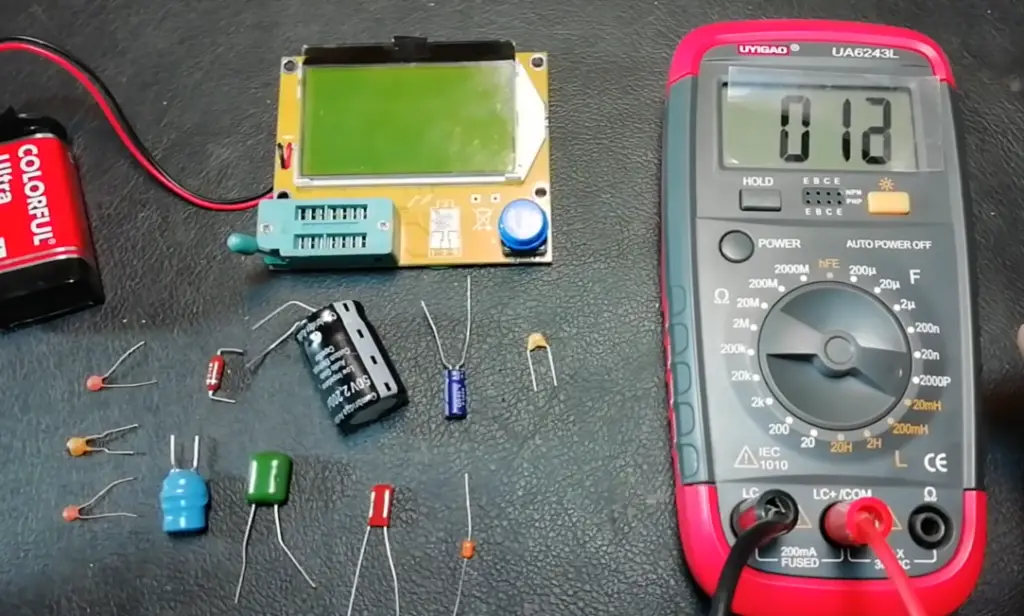### Input Terminals

LCR meters have input terminals where the component being measured is connected. These terminals are designed to accurately measure the component without causing damage.

## LCR Meter Uses

### Checking For Faulty Capacitors

An LCR meter is specifically designed to test the electrical properties of capacitors, inductors, and resistors.

These components are often found in electronic circuits and can become faulty over time. An LCR meter allows for accurate measurement of their critical values, such as capacitance, inductance, and resistance.

### Measuring Impedance

LCR meters also measure impedance, which is a combination of resistance, inductance, and capacitance in an AC circuit. Impedance can affect the performance of a circuit, so it’s important to keep track of this value as well.

### Checking If A Power Supply Is In Phase Or Not

LCR meters also have the capability to check if a power supply is in phase or not. This is important for ensuring that current flows through the circuit properly and efficiently.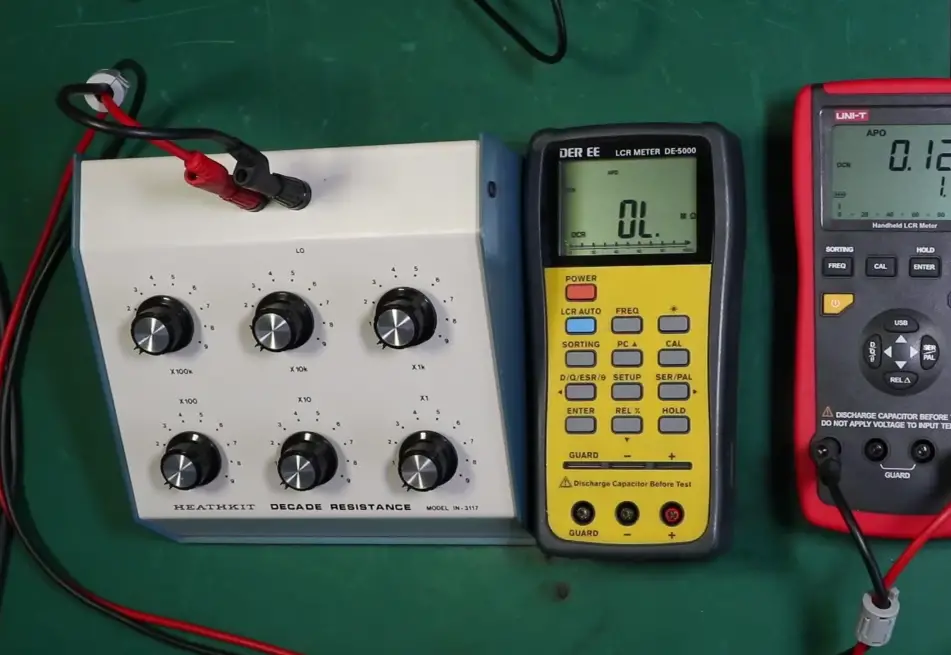### Examining An Oscillator Circuit

LCR meters can also be used to analyze an oscillator circuit. Oscillators generate AC signals, so it’s important to ensure that they are functioning properly and producing the desired frequency. LCR meters can measure the frequency of the output signal and determine if there are any issues with the circuit.

LCR meter, as the name implies, is a special measurement tool used to measure the parameters of electrical components in this certificate.

L refers to inductance, c refers to capacitance, and R refers to resistance. In addition, the LCR meter cannot measure anything else, and the inductance, resistance, or capacitance must be measured when the circuit is powered off. Because it is a special instrument, the measurement accuracy is high and the accuracy is strong.

In addition to measuring the inductance of the above resistance, capacitance, and inductance, the multimeter can also measure AC and DC voltages, currents, power frequency, etc. in different gears. Although it has more functions, the accuracy is not too accurate.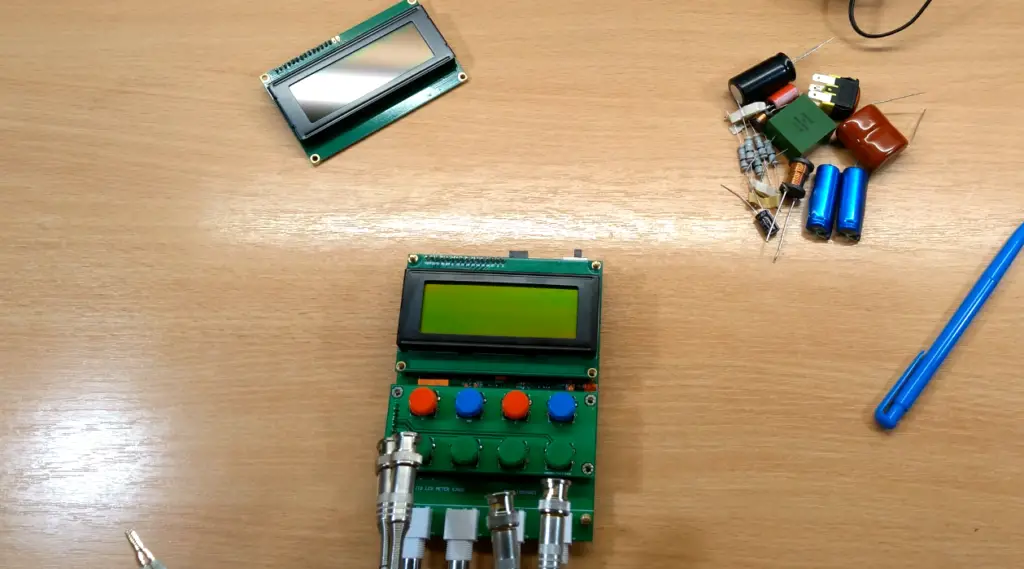Simply put, the LCR meter measures the intrinsic parameters of separate components, such as resistance, capacitance, etc., and it generally requires independent testing after the power is off. The multimeter is a multi-functional measuring instrument. It can test separate components and electrical signal characteristics. It also has some other measurement functions.

## What is the Difference Between a Multimeter and LCR Meter

### Measuring Units

One of the main differences between a multimeter and an LCR meter is the units they are able to measure. A multimeter is primarily used for measuring electrical values, such as voltage, current, and resistance.

On the other hand, an LCR meter is specifically designed for measuring inductance (L), capacitance (C), and resistance (R).

### Measurement Range

In addition to different measurement units, multimeters and LCR meters also have varying ranges of values that they can measure. Multimeters typically have a wider range of values that they can measure, while LCR meters tend to have more precise measurements within a limited range.

### Frequency Measurement

Another important difference is in their ability to measure frequency. Multimeters are not able to measure frequency, while LCR meters often have the capability to measure both AC and DC frequencies.

### Working Component

The working component of an LCR meter is also different from that of a multimeter. An LCR meter uses an AC excitation signal to measure the values, while a multimeter typically uses a DC excitation signal.

### General Use

In general, multimeters are more commonly used for basic electrical measurements in a variety of settings. LCR meters, on the other hand, are primarily used for testing and troubleshooting in industries such as electronics manufacturing and telecommunications. While both instruments have their own unique purposes, it is important to understand the differences between an LCR meter and a multimeter in order to choose the right tool for the job.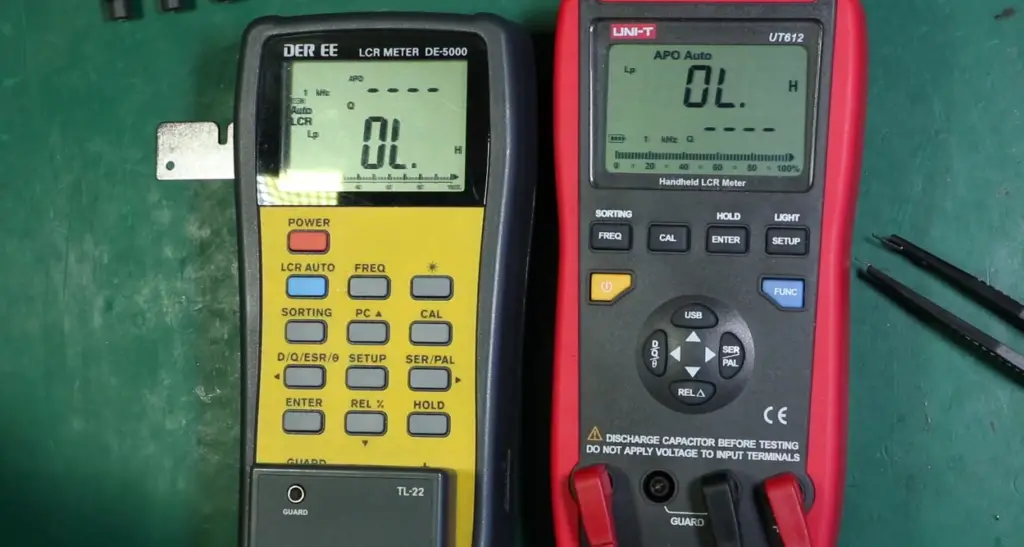## Are LCR Meters Better than Multimeters?

There is a common misconception that LCR meters are inherently better for measuring inductance, capacitance, and resistance than multimeters. While LCR meters often have higher accuracy and precision in these measurements, it ultimately depends on the specific model of each instrument.

One advantage of LCR meters is their ability to measure these components at various frequencies, allowing for more comprehensive testing. However, some high-end multimeters also have this capability.

In general, an LCR meter should be used when extremely precise measurements of inductance, capacitance, and resistance are needed.

A multimeter may be sufficient for basic testing and troubleshooting in most cases.

Ultimately, the best tool for the job depends on the specific application and the required level of accuracy. It is important for technicians to be familiar with both types of instruments and understand their capabilities in order to make an informed decision.

## FAQ

### Can the LCR meter be used as a multimeter?

No, the LCR meter is specifically designed to measure inductance (L), capacitance (C), and resistance (R). A multimeter, on the other hand, can measure a broader range of values including voltage, current, and resistance.

### What are some common uses for an LCR meter?

LCR meters are commonly used in electronic circuit design and testing, as well as quality control for manufactured components such as capacitors and inductors.

### Is an LCR meter necessary for all electronics projects?

It depends on the specific project. If your project requires precise measurements of inductance, capacitance, or resistance, then an LCR meter would be necessary. However, if your project only requires measuring voltage or current, a multimeter would suffice.

It is also important to note that LCR meters tend to be more expensive than multimeters, so it may not always be practical to purchase one for every project. In these cases, a multimeter can often provide adequate measurements for inductance and capacitance as well.

### How accurate are LCR meters?

LCR meters are highly accurate, with some models capable of measuring impedance down to 0.1%.

This level of precision is essential for testing circuit components such as capacitors and inductors.

### Can a multimeter measure impedance?

While multimeters can measure resistance, they are not capable of accurately measuring the complex impedance of circuit components. This makes LCR meters the preferred tool for these measurements.

### Why LRC meter is important?

An LCR meter, or inductance, capacitance, and resistance meter is a specialized type of electronic test equipment used to measure the values of components in electronic circuits. It is important for measuring the quality and performance of components such as capacitors and inductors.

On the other hand, a multimeter is a versatile tool that can measure multiple values such as voltage, current, and resistance. However, it is not as accurate or precise as an LCR meter when it comes to measuring inductance, capacitance, and resistance.

When working with electronic circuits, it is often necessary to have both an LCR meter and a multimeter on hand.

### Can you use an LCR meter in the circuit?

No, an LCR meter is not designed to be used while a circuit is powered. It must be disconnected from the circuit in order to take measurements.

### How do you read an LCR meter?

An LCR meter measures inductance (L), capacitance (C), and resistance (R) in circuit components. It generally has three leads: one labeled “common,” one labeled “measure,” and one labeled either “inductance” or “capacitance.” To measure a component, you would connect the common lead to one side of the component and the appropriate measurement lead (either inductance or capacitance) to the other side. The reading displayed on the meter is the value of that component.

### How do I choose an LCR meter?

When choosing an LCR meter, it is important to consider the range of values that you will need to measure. Different meters have different measurement capabilities, so make sure to choose one with a wide enough range for your needs. Additionally, some LCR meters also have additional features such as testing frequency and quality factor (Q) measurements. Consider whether or not these extra features would be useful for your applications.

It is also important to consider the accuracy and resolution of the LCR meter. Higher accuracy and resolution will result in more precise measurements but may come at a higher price point. Determine what level of precision is necessary for your applications and choose an LCR meter accordingly.

### What is the difference between Impedance Analyzer and LCR meter?

An Impedance Analyzer is a type of LCR meter that measures not only inductance, capacitance, and resistance, but also the component’s impedance.

Impedance is a measure of the combined effects of resistance, inductance, and capacitance on the flow of alternating current in a circuit. Thus, an Impedance Analyzer can provide more comprehensive information about circuit components than a standard LCR meter.

In contrast, a multimeter measures electrical properties such as voltage, current, and resistance. It does not have the capability to measure inductance or capacitance as an LCR meter does. Additionally, a multimeter cannot measure impedance. Thus, for measuring the characteristics of circuit components, an LCR meter (or Impedance Analyzer) is the preferred tool. However, a multimeter can still be useful for general electrical testing and troubleshooting.

Some LCR meters do have an option for voltage and current display. An important function that many modern LCR meters have is measuring the equivalent series resistance of capacitors or inductors in a circuit without having to remove the components.

For proper testing, this is an important feature. Overall, in more cases, LCRs can prove to be more useful than multimeters.

### How do you measure inductance with an LCR meter?

An LCR meter specifically measures inductance, capacitance, and resistance. To measure inductance, the device sends an alternating current through the component being tested and measures the resulting voltage drop.

On the other hand, a multimeter can also measure these values but is not as precise or accurate as an LCR meter. Additionally, a multimeter can also measure other values such as voltage and current.

So when choosing between an LCR meter and a multimeter for measuring inductance, it is best to use an LCR meter for more precise and accurate results.

### Can a capacitor be tested while in the circuit?

An LCR meter, or inductance, capacitance, and resistance meter are specifically designed to measure these values in the circuit. It also typically has higher accuracy and resolution than a multimeter.

However, a multimeter can still be used to measure these values as well. But because it is not specifically designed for this purpose, its accuracy and resolution may not be as high. In addition, it cannot directly measure inductance in a circuit without additional equipment.

### What are the advantages of a digital LCR bridge?

While a multimeter is able to measure basic electrical values such as voltage, current, and resistance, an LCR meter offers more precise measurements of inductance (L), capacitance (C), and resistance (R). It also has the ability to calculate the quality factor or “Q” value of an electronic component. This can be crucial when designing high-frequency circuits where small changes in component values can have significant impacts on performance.

Another advantage of using an LCR meter is its ability to measure at different frequencies. This allows for more accurate readings as the behavior of some components may change with varying frequencies.

Overall, using a digital LCR bridge can provide greater accuracy and flexibility in measuring complex electronic components.

### How do you measure inductance without an LCR meter?

One way to measure inductance without an LCR meter is by using a multimeter. However, it is important to note that a multimeter may not be as accurate or precise as an LCR meter.

To measure inductance with a multimeter, set the device to the correct function (usually labeled as “IND” or “L”). Then, connect one lead of the multimeter to the component’s positive terminal and the other lead to the negative terminal. The reading on the display will show the inductance in microhenries (µH).

### What is the LCR meter block diagram?

The LCR meter block diagram consists of a signal source, an impedance converter, and a measurement circuit. The signal source generates an AC signal that is applied to the component under test. The impedance converter then converts the complex impedance of the component into separate resistance (R), inductance (L), and capacitance (C) values. These values are then measured by the measurement circuit and displayed on the LCR meter’s screen.

### Is an LCR meter useful in measuring the conductance and susceptance?

While a multimeter can also measure these components, an LCR meter is specifically designed for measuring inductance (L), capacitance (C), and resistance (R). This makes these more accurate and precise in these measurements.

Additionally, an LCR meter often has the ability to measure impedance, quality factor, and phase angle – capabilities that a standard multimeter does not have.

So, while a multimeter may be able to give you a rough idea of the conductance and susceptance values, an LCR meter is the preferred tool for obtaining more accurate readings.

### What is the disadvantage of using a digital multimeter?

One disadvantage of using a digital multimeter is that it typically cannot measure impedance, which is one of the key measurements for an LCR meter. Additionally, a multimeter may not be able to accurately measure the true value of inductance or capacitance due to its limited frequency range.

In contrast, an LCR meter is specifically designed for measuring impedance, inductance, and capacitance. It also often has a wider frequency range, allowing for more accurate measurements in these areas.

### What are the disadvantages of an analog multimeter?

First, an analog multimeter does not have the precision or accuracy of an LCR meter. This can lead to unreliable readings and potential errors in measurements.

Additionally, an analog multimeter cannot measure impedance or reactance. It also cannot perform complex measurement tasks such as testing for equivalent series resistance (ESR) or quality factor (Q).

In contrast, an LCR meter is specifically designed for measuring inductance, capacitance, and resistance with high precision and accuracy. It also has the ability to measure impedance and reactance, as well as perform more advanced measurement tasks.

### How do you know if an inductor is bad?

One option is a multimeter, which can measure voltage, current, and resistance. However, for more specific measurements related to inductance (L), capacitance (C), and resistance (R), an LCR meter is necessary.

An LCR meter measures these values with higher precision than a multimeter. It also often includes additional features such as frequency measurement and impedance analysis.

So when it comes to testing components in electronic circuits, having both an LCR meter and a multimeter in your toolkit provides the most comprehensive solution.

### Does inductance change with frequency?

While a multimeter can measure inductance, it typically only measures at a single frequency. An LCR meter, on the other hand, is capable of measuring at multiple frequencies and can also show how the inductance changes with varying frequencies. This makes an LCR meter more useful for analyzing components such as capacitors and inductors.

Additionally, an LCR meter often has higher accuracy and precision than a multimeter when measuring components with low resistance values.

Overall, if you need to measure or analyze the characteristics of components like capacitors and inductors, an LCR meter would be the better tool for the job. However, for general electrical measurements such as voltage and resistance, a multimeter would suffice.